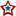MN-Sen: Recount Regression, Day 3

For a more complete explanation of the process used, please refer to my initial post on the subject.

Recap of today's official result:

Recount      Original
Coleman:   751898   -    752438 =  -540
Franken:   723378   -    723818 =  -440

Franken Net Today: 14 (43 yesterday, 43 day before)
Total Franken Net: 100
Franken Deficit:  115

The following two graphs depict the number of votes gained or lost with respect to the total votes recorded for each precinct that has completed their recount. Each dot represents the change between the originally certified result and the post recount tally in a given precinct with x number of total votes:And the current functions used in conjunction with the regression:Now again using those functions, the following two graphs further illustrate the regression interpolation. The graph below illustrates the regression on precincts whose vote totals are less than 3,494; the largest precinct that has completed a recount. The dotted lines represent the first day's regression, while the dashed lines represent yesterday's. The thin lines depict the linear regression while the thicker curvy lines present a quartic regression. The black bars emanating from the x axis represent the number of precincts, statewide with x number of total votes. There are a few straggler precincts between 2600 and 6621 that are not depicted due to the resolution of the graph.The graph below goes further and fits the regression onto all 4,130 precincts statewide; this forces 32,186 additional votes, that lie in precincts that surpass the 3,494 vote threshold, into the predetermined trend.Using the previous two graphs, and the functions they represent, a projection can be made for the cases covered. The first case simply includes precincts with 3,494 or less, while precincts above and beyond that figure are entirely ignored. For each precinct, the total vote total is taken and applied to the listed function for each candidate. The result is then added to that candidate's sum, and the next precinct is calculated. This process is done using precinct results from the final certification.

Coleman Gain: -832.977997236315 (+/- 24)
Franken Gain: -681.983934516065 (+/- 20)

Franken Net: 150.994062720251 (+/- 44)

Franken falls short of the pre-recount deficit by about 65 votes, with 32,186 votes entirely ignored and the challenges left uncounted. If those additional 32,186 votes are applied to the process, Franken adds an additional vote:

Coleman Gain: -844.072810341355 (+/- 103)
Franken Gain: -691.688480481963 (+/- 97)

Franken Net: 152.384329859392 (+/- 200)

As of right now (10:24 PM CT 11/21), after 60.86% of all votes have been recounted. Check back tomorrow for the day 4 result, if there is one, its Saturday and I don't know if they continue counting.

0 Response(s) to MN-Sen: Recount Regression, Day 3(Word Verification)